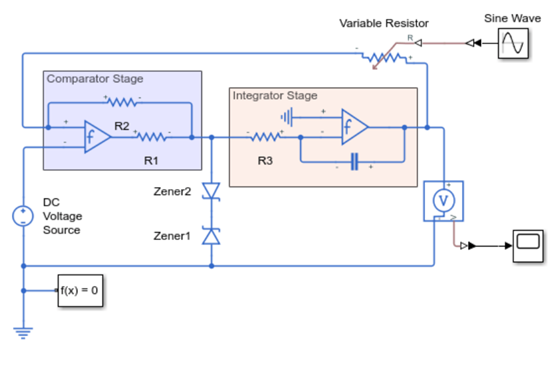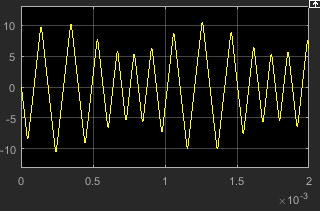## Build and Simulate a Simple Circuit

In this example, you model a triangle wave generator using Simscape™ Electrical™ blocks and custom Simscape Electrical blocks, and then look at the voltage at the wave generator output.

You use a classic circuit configuration consisting of an integrator and a noninverting amplifier to generate the triangle wave, and use datasheets to specify block parameters. For more information, see Parameterizing Blocks from Datasheets.

To see the completed model, open the Triangle Wave Generator example.

### Select Blocks to Represent System Components

First, you select the blocks to represent the input signal, the triangle wave generator, and the output signal display.

You model the triangle wave generator with a set of physical blocks. The wave generator consists of:

• Two operational amplifier blocks

• Resistors and a capacitor that work with the operational amplifiers to create the integrator and noninverting amplifier

• Simulink-PS Converter and PS-Simulink Converter blocks whose function is to bridge the physical part of the model, which uses physical signals, and the rest of the model, which uses Simulink® signals.

You have a manufacturer datasheet for the two operational amplifiers you want to model. Later in the example, you use the datasheet to parameterize the Simscape Electrical Band-Limited Op-Amp block.

The following table describes the role of the blocks that represent the system components.

Block

Description

Sine Wave

Generates a sinusoidal signal that controls the resistance of the Variable Resistor block

Scope

Displays the triangular output wave

Solver Configuration

Defines solver settings that apply to all physical modeling blocks

CapacitorWorks with an operational amplifier and resistor block to create the integrator
ResistorWorks with the operational amplifier and capacitor blocks to create the integrator and noninverting amplifier
Variable ResistorSupplies a time-varying resistance that adjusts the gain of the integrator, which in turn varies the frequency and amplitude of the generated triangular wave
DC Voltage Source

Generates a DC reference signal for the operational amplifier block of the noninverting amplifier

Voltage Sensor

Converts the electrical voltage at the output of the integrator into a physical signal proportional to the current

Electrical Reference

Provides the electrical ground

Band-Limited Op-AmpWorks with the capacitor and resistor to create an integrator and a noninverting amplifier
DiodeLimits the output of the Band-Limited Op-Amp block, to make the output waveform independent of supply voltage

### Build the Model

Create a Simulink model, add blocks to the model, and connect the blocks.

1. Create a new model.

2. Add to the model the blocks listed in this table. The Library Path column of the table specifies the hierarchical path to each block.

Block

Library Path

Quantity

1

1

1

Solver ConfigurationSimscape > Utilities

1

1

CapacitorSimscape > Foundation Library > Electrical > Electrical Elements

1

ResistorSimscape > Foundation Library > Electrical > Electrical Elements

3

Variable ResistorSimscape > Foundation Library > Electrical > Electrical Elements

1

Electrical ReferenceSimscape > Foundation Library > Electrical > Electrical Elements

2

DC Voltage SourceSimscape > Foundation Library > Electrical > Electrical Sources

1

Voltage SensorSimscape > Foundation Library > Electrical > Electrical Sensors

1

Band-Limited Op-Amp

Simscape > Electrical > Integrated Circuits

2

Diode

Simscape > Electrical > Semiconductor & Converters

2

Note

You can use the Simscape function `ssc_new` with a domain type of `electrical` to create a Simscape model that contains these blocks:

• Scope

• Solver Configuration

• Electrical Reference

3. Rename and connect the blocks as shown in the diagram. The blocks in the triangle wave generator circuit are organized in two stages. The Comparator Stage contains a comparator constructed from a Band-Limited Op-Amp block and two Resistor blocks. The Integrator Stage contains an integrator constructed from another Band-Limited Op-Amp block, a Resistor, a Capacitor, and Electrical Reference.### Specify Model Parameters

Specify these parameters to represent the behavior of the system components:

#### Model Setup Parameters

These blocks specify model information that is not specific to a particular block:

• Solver Configuration

• Electrical Reference

As with Simscape models, you must include a Solver Configuration block in each topologically distinct physical network. This example has a single physical network, so use one Solver Configuration block with the default parameter values.

You must include an Electrical Reference block in each Simscape Electrical network. This block does not have any parameters.

#### Input Signal Parameters

Generate the sinusoidal control signal using the Sine Wave block.

Set the Sine Wave block parameters as follows:

• Amplitude`0.5e4`

• Bias`1e4`

• Frequency`pi/5e-4`

#### Triangle Wave Generator Parameters

Configure the blocks modeling the physical system that generates the triangle wave:

• Integrator stage — Band-Limited Op-Amp, Capacitor, and Resistor block R3

• Comparator stage — Band-Limited Op-Amp1, Resistor blocks R1 and R2

• Variable Resistor

• Diode and Diode1

• Simulink-PS Converter and PS-Simulink Converter blocks that bridge the physical part of the model and the Simulink part of the model.

1. Accept the default parameters for the Simulink-PS Converter block. These parameters establish the units of the physical signal at the block output such that they match the expected default units of the Variable Resistor block input.

2. Set the two Band-Limited Op-Amp block parameters for the LM7301 device with a +–20V power supply:

• The datasheet gives the gain as 97 dB, which is equivalent to 10 ^ ( 97 / 20 ) = 7.1e4. Set the Gain, A parameter to `7.1e4`.

• The datasheet gives input resistance as 39 Mohms. Set Input resistance, Rin to `39e6`.

• Set Output resistance, Rout to `0` ohms. The datasheet does not quote a value for Rout, but the term is insignificant compared to the output resistor that it drives.

• Set minimum and maximum output voltages to –20 V and +20 V, respectively.

• The datasheet gives the maximum slew rate as 1.25 V/μs. Set the Maximum slew rate, Vdot parameter to `1.25e6` V/s.

• Set the bandwidth to `4e6`.

3. Set the two Diode block parameters for a 4.3 V zener diode. To model a BZX384-B4V3, set block parameters as follows:

• On the Main tab, set Diode model to `Piecewise Linear`. This selects a simplified Zener diode model that is more than adequate to test the correct operation of this circuit.

• Leave the Forward voltage as 0.6 V — this is a typical value for most diodes.

• The datasheet gives the forward current as 250 mA when the forward voltage is 1V. So that the Diode block matches this, set the On resistance to (1 V – 0.6 V) / 250 mA = `1.6` ohms.

• The datasheet gives the reverse leakage current as 3 μA at a reverse voltage of 1 V. Therefore, set the Off conductance to 3 μA / 1 V = `3e-6` S.

• The datasheet gives the reverse voltage as 4.3 V. On the Breakdown tab, set the Reverse breakdown voltage Vz to `4.3` V.

• Set the Zener resistance Rz to a suitably small number. The datasheet quotes the Zener voltage for a reverse current of 5 mA. For the Diode block to be representative of the real device, the simulated reverse voltage should be close to 4.3V at 5mA. As Rz tends to zero, the reverse breakdown voltage tends to Vz regardless of current, as the voltage-current gradient becomes infinite. However, for good numerical properties, Rz must not be made too small. If, say, you allow a 0.01 V error on the Zener voltage at 5 mA, then Rz is 0.01 V / 5 mA = 2 ohms. Set the Zener resistance parameter to this value.

4. The Voltage Sensor block does not have any parameters.

5. Accept the default parameters for the Variable Resistor block. These parameters establish the units of the physical signal at the block output such that they match the expected default units of the Variable Resistor block input.

6. Set the Capacitor block parameters as follows:

• Capacitance`2.5e-9`

• Capacitor voltage`0.08`

This value starts the oscillation in the feedback loop. It is found in the Variables tab.

• Series resistance`0`

7. Set the DC Voltage Source block Constant voltageparameter to `0`.

8. Set the Resistor R3 block Resistance parameter to `10000`.

9. Set the Resistor R1 block Resistance parameter to `1000`.

10. Set the Resistor R2 block Resistance parameter to `10000`.

11. Accept the default parameters for the PS-Simulink Converter block. These parameters establish the units of the physical signal at the block output such that they match the expected default units of the Scope block input.

#### Signal Display Parameters

Specify the parameters of the Scope block to display the triangular output signal.

Double-click the Scope block and then click the View > Configuration Properties to open the Scope Configuration Properties dialog box. On the Logging tab, clear the Limit data points to last check box.

### Configure the Solver Parameters

Configure the solver parameters to use a continuous-time solver.Simscape Electrical models only run with a continuous-time solver when the Simscape Solver Configuration block has its Local Solver parameter cleared. You also change the simulation end time, tighten the relative tolerance for a more accurate simulation, and remove the limit on the number of simulation data points Simulink saves.

1. In the model window, select Modeling > Model Settings to open the Configuration Parameters dialog box.

2. In the Solver category in the tree on the left side of the dialog box:

• Enter `2000e-6` for the Stop time parameter value.

• Select `ode23t (Mod. stiff/Trapezoidal)` from the Solver list.

• Enter `4e-5` for the Max step size parameter value.

• Enter `1e-6` for the Relative tolerance parameter value.

3. In the Data Import/Export category in the Select tree, clear the Limit data points to last check box.

4. Click OK.

### Simulate Model and Analyze Results

Run the simulation and plot the results.

In the model window, select Simulation > Run to run the simulation.

To view the triangle wave in the Scope window, double-click the Scope block. You can do this before or after you run the simulation.

The following plot shows the voltage waveform. As the resistance of the Variable Resistor block increases, the amplitude of the output waveform increases and the frequency decreases.

Triangle Waveform Voltage